Courses

# Digital Electronics NAT Level - 2

## 10 Questions MCQ Test Topic wise Tests for IIT JAM Physics | Digital Electronics NAT Level - 2

Description
This mock test of Digital Electronics NAT Level - 2 for IIT JAM helps you for every IIT JAM entrance exam. This contains 10 Multiple Choice Questions for IIT JAM Digital Electronics NAT Level - 2 (mcq) to study with solutions a complete question bank. The solved questions answers in this Digital Electronics NAT Level - 2 quiz give you a good mix of easy questions and tough questions. IIT JAM students definitely take this Digital Electronics NAT Level - 2 exercise for a better result in the exam. You can find other Digital Electronics NAT Level - 2 extra questions, long questions & short questions for IIT JAM on EduRev as well by searching above.
*Answer can only contain numeric values
QUESTION: 1

### The minimum number of NAND gates required to implement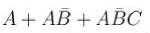?

Solution: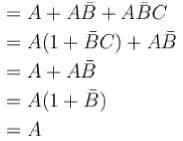Hence, minimum number of NAND gates required to implement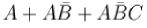is zero.

*Answer can only contain numeric values
QUESTION: 2

### The output of the circuit in the figure is equal to.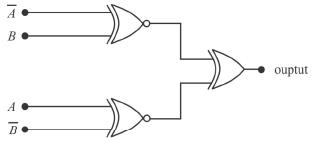Solution: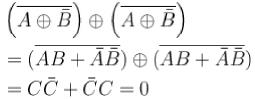*Answer can only contain numeric values
QUESTION: 3

### The logic function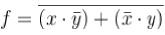for x = 1, y= 0 gives?

Solution: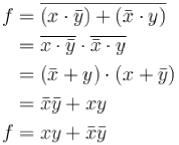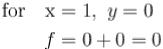*Answer can only contain numeric values
QUESTION: 4

What will be the output of the logic gate formed by the circuit drawn below for inputs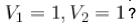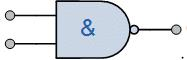Solution:

The above figure behaves as a NAND gate, hence for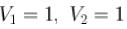and output = 0

*Answer can only contain numeric values
QUESTION: 5

What is the output of the Boolean expression for the output of the logic circuit drawn below for A = B = C = 1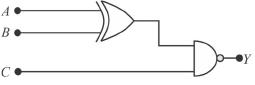Solution: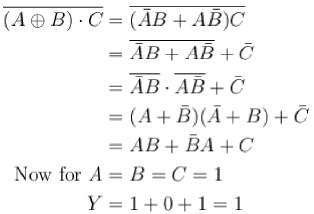*Answer can only contain numeric values
QUESTION: 6

What is the output for X = 1, Y = 0 for the logic circuit drawn below :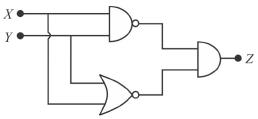Solution:

The truth table for the above circuit is.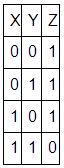Hence, for X = 1, Y = 0, output = 1

*Answer can only contain numeric values
QUESTION: 7

The Boolean expression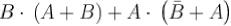can be realized by how many minimum number of gates?

Solution: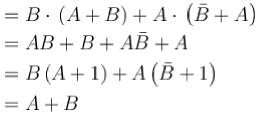Hence one two input AND gate is required.
∴ Minimum number of gate = 1.

*Answer can only contain numeric values
QUESTION: 8

Find the output for A = 1, B = 0, C = 1.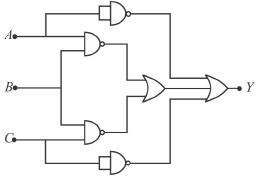Solution: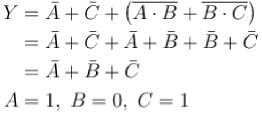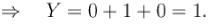*Answer can only contain numeric values
QUESTION: 9

Assuming that only the X and Y logic inputs are available and their complements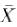and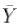are not available, what is the minimum number of two-input NAND gates require to implement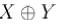Solution: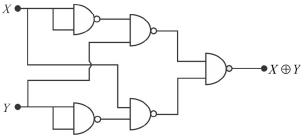*Answer can only contain numeric values
QUESTION: 10

Consider following gate network which of the following gate is redundant write the gate number____ ?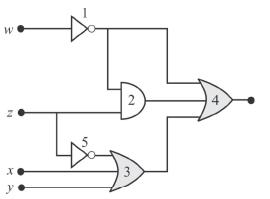Solution:

Before gate Number 2 being remove, output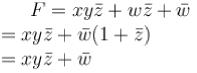⇒ gate number 2 is redundant.